# Days In Green (DIG): Forecasting the life of a healthy service

Jun. 26, 2014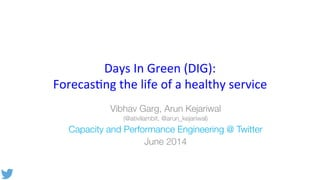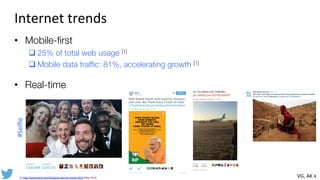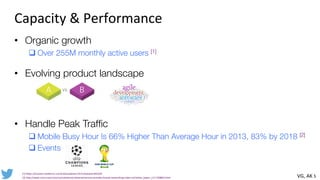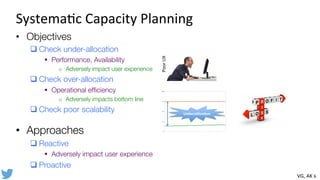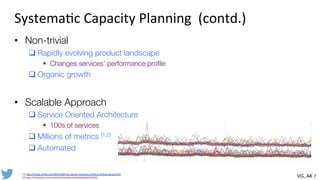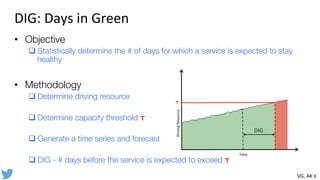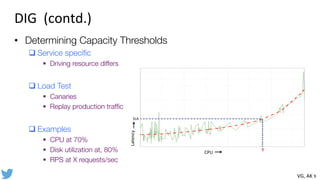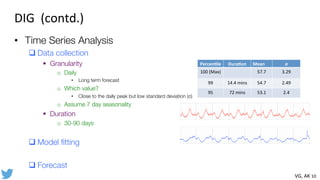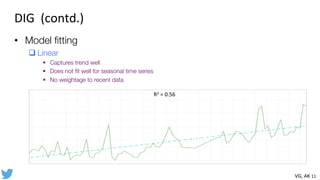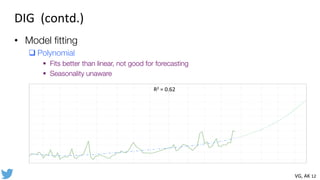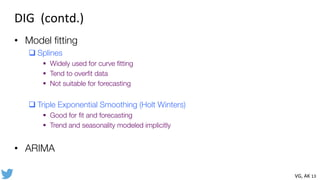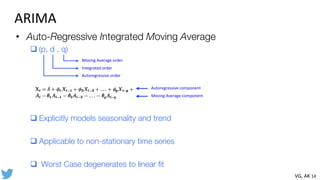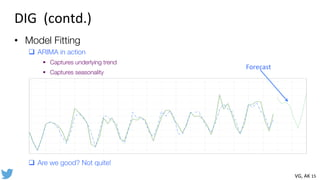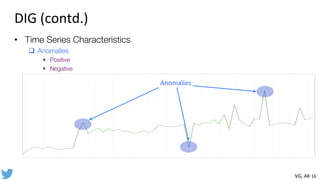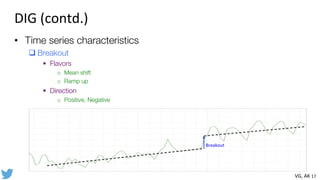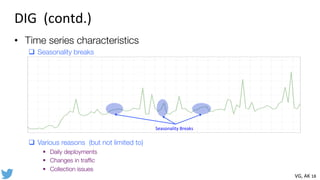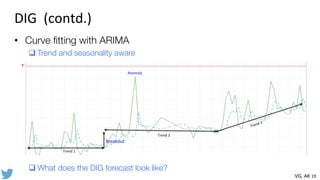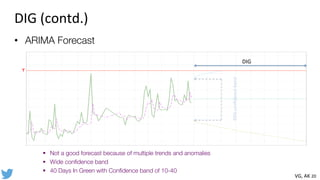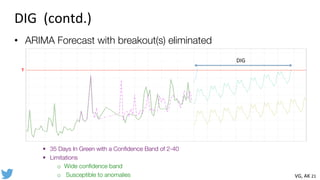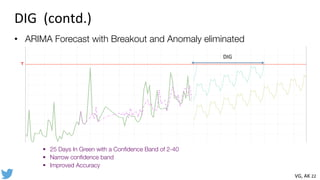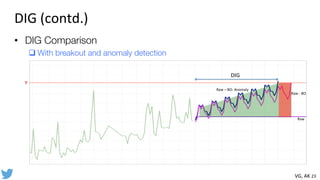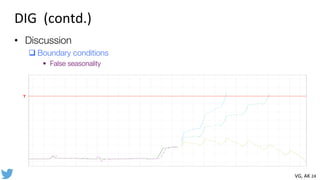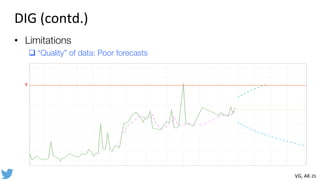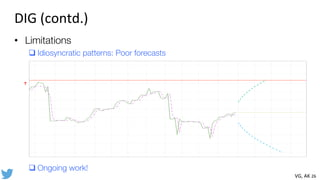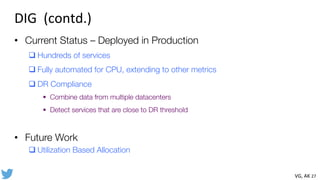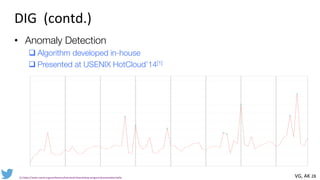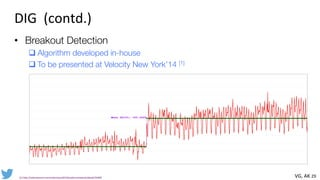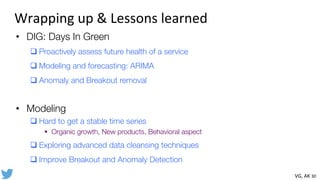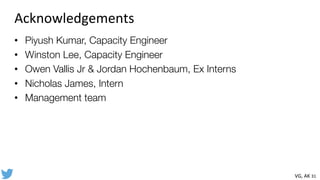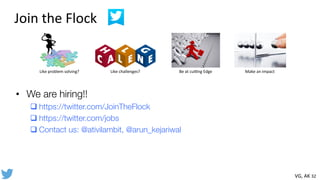1 of 32

### Days In Green (DIG): Forecasting the life of a healthy service

• 1. Days  In  Green  (DIG):     Forecas1ng  the  life  of  a  healthy  service   Vibhav Garg, Arun Kejariwal (@ativilambit, @arun_kejariwal) Capacity and Performance Engineering @ Twitter June 2014
• 2. #WorldCup     hBp://www.newsweek.com/twiBer-­‐us-­‐soccer-­‐what-­‐radio-­‐was-­‐baseball-­‐256336  (June  2014)   
• 3.   hBp://www.telegraph.co.uk/technology/twiBer/10912738/Wimbledon-­‐goes-­‐digital-­‐with-­‐TwiBer.html  (June  2014)      #Wimbledon2014
• 4. Internet  trends   •  Mobile-ﬁrst q 25% of total web usage  q Mobile data trafﬁc: 81%, accelerating growth  •  Real-time   hBp://www.kpcb.com/ﬁle/kpcb-­‐internet-­‐trends-­‐2014  (May  2014)   VG,  AK  4   #Selﬁe
• 5. Capacity  &  Performance   •  Organic growth q Over 255M monthly active users  •  Evolving product landscape •  Handle Peak Trafﬁc q Mobile Busy Hour Is 66% Higher Than Average Hour in 2013, 83% by 2018  q Events   hBps://investor.twiBerinc.com/releasedetail.cfm?releaseid=843245     hBp://www.cisco.com/c/en/us/solu1ons/collateral/service-­‐provider/visual-­‐networking-­‐index-­‐vni/white_paper_c11-­‐520862.html   VG,  AK  5
• 6. Systema1c  Capacity  Planning   •  Objectives q Check under-allocation §  Performance, Availability o  Adversely impact user experience q Check over-allocation §  Operational efﬁciency o  Adversely impacts bottom line q Check poor scalability •  Approaches q Reactive §  Adversely impact user experience q Proactive Poor  UX   Underu'liza'on   VG,  AK  6
• 7. Systema1c  Capacity  Planning    (contd.)   •  Non-trivial q Rapidly evolving product landscape §  Changes services’ performance proﬁle q Organic growth •  Scalable Approach q Service Oriented Architecture §  100s of services q Millions of metrics [1,2] q Automated   hBp://strata.oreilly.com/2013/09/how-­‐twiBer-­‐monitors-­‐millions-­‐of-­‐1me-­‐series.html     hBp://strataconf.com/strata2014/public/schedule/detail/32431   VG,  AK  7
• 8. DIG:  Days  in  Green   •  Objective q Statistically determine the # of days for which a service is expected to stay healthy •  Methodology q Determine driving resource q Determine capacity threshold T q Generate a time series and forecast q DIG - # days before the service is expected to exceed T VG,  AK  8   Time   Driving  Resource   DIG   T
• 9. DIG    (contd.)   •  Determining Capacity Thresholds q Service speciﬁc §  Driving resource differs q Load Test §  Canaries §  Replay production trafﬁc q Examples §  CPU at 70% §  Disk utilization at, 80% §  RPS at X requests/sec VG,  AK  9   SLA   T   CPU   Latency
• 10. DIG    (contd.)   •  Time Series Analysis q Data collection §  Granularity o  Daily •  Long term forecast o  Which value? •  Close to the daily peak but low standard deviation (σ) o  Assume 7 day seasonality §  Duration o  30-90 days q Model ﬁtting q Forecast VG,  AK  10   Percen'le   Dura'on   Mean   σ   100  (Max)   57.7   3.29   99   14.4  mins   54.7   2.49   95   72  mins   53.1   2.4
• 11. DIG    (contd.)   •  Model ﬁtting q Linear §  Captures trend well §  Does not ﬁt well for seasonal time series §  No weightage to recent data VG,  AK  11   R2  =  0.56
• 12. DIG    (contd.)   •  Model ﬁtting q Polynomial §  Fits better than linear, not good for forecasting §  Seasonality unaware VG,  AK  12   R2  =  0.62
• 13. DIG    (contd.)   •  Model ﬁtting q Splines §  Widely used for curve ﬁtting §  Tend to overﬁt data §  Not suitable for forecasting q Triple Exponential Smoothing (Holt Winters) §  Good for ﬁt and forecasting §  Trend and seasonality modeled implicitly •  ARIMA VG,  AK  13
• 14. ARIMA   •  Auto-Regressive Integrated Moving Average q (p, d , q) q Explicitly models seasonality and trend q Applicable to non-stationary time series q  Worst Case degenerates to linear ﬁt Autoregressive  component   Moving  Average  component   Moving  Average  order   Integrated  order   Autoregressive  order   VG,  AK  14
• 15. DIG    (contd.)   •  Model Fitting q  ARIMA in action §  Captures underlying trend §  Captures seasonality q  Are we good? Not quite! VG,  AK  15   Forecast
• 16. •  Time Series Characteristics q  Anomalies §  Positive §  Negative VG,  AK  16   Anomalies   DIG  (contd.)
• 17. Breakout   •  Time series characteristics q Breakout §  Flavors o  Mean shift o  Ramp up §  Direction o  Positive, Negative DIG  (contd.)   VG,  AK  17
• 18. •  Time series characteristics q  Seasonality breaks q  Various reasons (but not limited to) §  Daily deployments §  Changes in trafﬁc §  Collection issues Seasonality  Breaks   VG,  AK  18   DIG    (contd.)
• 19. VG,  AK  19   •  Curve ﬁtting with ARIMA q Trend and seasonality aware q What does the DIG forecast look like? Trend  1   Trend  2   DIG    (contd.)   Trend  3   Anomaly   T   Breakout
• 20. DIG  (contd.)   •  ARIMA Forecast §  Not a good forecast because of multiple trends and anomalies §  Wide conﬁdence band §  40 Days In Green with Conﬁdence band of 10-40 VG,  AK  20   95%  conﬁdence  band   T   DIG
• 21. •  ARIMA Forecast with breakout(s) eliminated §  35 Days In Green with a Conﬁdence Band of 2-40 §  Limitations o  Wide conﬁdence band o  Susceptible to anomalies VG,  AK  21   DIG    (contd.)   T   DIG
• 22. •  ARIMA Forecast with Breakout and Anomaly eliminated §  25 Days In Green with a Conﬁdence Band of 2-40 §  Narrow conﬁdence band §  Improved Accuracy VG,  AK  22   DIG    (contd.)   T   DIG
• 23. •  DIG Comparison q With breakout and anomaly detection DIG  (contd.)   VG,  AK  23   DIG   T   Raw   Raw  -­‐  BO   Raw  –  BO-­‐  Anomaly
• 24. DIG    (contd.)   VG,  AK  24   •  Discussion q Boundary conditions §  False seasonality T
• 25. DIG  (contd.)   •  Limitations q “Quality” of data: Poor forecasts VG,  AK  25   T
• 26. •  Limitations q Idiosyncratic patterns: Poor forecasts q Ongoing work! VG,  AK  26   DIG  (contd.)   T
• 27. DIG    (contd.)   VG,  AK  27   •  Current Status – Deployed in Production q Hundreds of services q Fully automated for CPU, extending to other metrics q DR Compliance §  Combine data from multiple datacenters §  Detect services that are close to DR threshold •  Future Work q Utilization Based Allocation
• 28. DIG    (contd.)   VG,  AK  28   •  Anomaly Detection q Algorithm developed in-house q Presented at USENIX HotCloud’14   hBps://www.usenix.org/conference/hotcloud14/workshop-­‐program/presenta1on/vallis
• 29. Mean Shift: 105.466%Mean Shift: 105.466%Mean Shift: 105.466%Mean Shift: 105.466%Mean Shift: 105.466%Mean Shift: 105.466%Mean Shift: 105.466%Mean Shift: 105.466%Mean Shift: 105.466%Mean Shift: 105.466%Mean Shift: 105.466%Mean Shift: 105.466%Mean Shift: 105.466%Mean Shift: 105.466%Mean Shift: 105.466%Mean Shift: 105.466%Mean Shift: 105.466%Mean Shift: 105.466%Mean Shift: 105.466%Mean Shift: 105.466%Mean Shift: 105.466%Mean Shift: 105.466%Mean Shift: 105.466%Mean Shift: 105.466%Mean Shift: 105.466%Mean Shift: 105.466%Mean Shift: 105.466%Mean Shift: 105.466%Mean Shift: 105.466%Mean Shift: 105.466%Mean Shift: 105.466%Mean Shift: 105.466%Mean Shift: 105.466%Mean Shift: 105.466%Mean Shift: 105.466%Mean Shift: 105.466%Mean Shift: 105.466%Mean Shift: 105.466%Mean Shift: 105.466%Mean Shift: 105.466%Mean Shift: 105.466%Mean Shift: 105.466%Mean Shift: 105.466%Mean Shift: 105.466%Mean Shift: 105.466%Mean Shift: 105.466%Mean Shift: 105.466%Mean Shift: 105.466%Mean Shift: 105.466%Mean Shift: 105.466%Mean Shift: 105.466%Mean Shift: 105.466%Mean Shift: 105.466%Mean Shift: 105.466%Mean Shift: 105.466%Mean Shift: 105.466%Mean Shift: 105.466%Mean Shift: 105.466%Mean Shift: 105.466%Mean Shift: 105.466%Mean Shift: 105.466%Mean Shift: 105.466%Mean Shift: 105.466%Mean Shift: 105.466%Mean Shift: 105.466%Mean Shift: 105.466%Mean Shift: 105.466%Mean Shift: 105.466%Mean Shift: 105.466%Mean Shift: 105.466%Mean Shift: 105.466%Mean Shift: 105.466%Mean Shift: 105.466%Mean Shift: 105.466%Mean Shift: 105.466%Mean Shift: 105.466%Mean Shift: 105.466%Mean Shift: 105.466%Mean Shift: 105.466%Mean Shift: 105.466%Mean Shift: 105.466%Mean Shift: 105.466%Mean Shift: 105.466%Mean Shift: 105.466%Mean Shift: 105.466%Mean Shift: 105.466%Mean Shift: 105.466%Mean Shift: 105.466%Mean Shift: 105.466%Mean Shift: 105.466%Mean Shift: 105.466%Mean Shift: 105.466%Mean Shift: 105.466%Mean Shift: 105.466%Mean Shift: 105.466%Mean Shift: 105.466%Mean Shift: 105.466%Mean Shift: 105.466%Mean Shift: 105.466%Mean Shift: 105.466%Mean Shift: 105.466%Mean Shift: 105.466%Mean Shift: 105.466%Mean Shift: 105.466%Mean Shift: 105.466%Mean Shift: 105.466%Mean Shift: 105.466%Mean Shift: 105.466%Mean Shift: 105.466%Mean Shift: 105.466%Mean Shift: 105.466%Mean Shift: 105.466%Mean Shift: 105.466%Mean Shift: 105.466%Mean Shift: 105.466%Mean Shift: 105.466%Mean Shift: 105.466%Mean Shift: 105.466%Mean Shift: 105.466%Mean Shift: 105.466%Mean Shift: 105.466%Mean Shift: 105.466%Mean Shift: 105.466%Mean Shift: 105.466%Mean Shift: 105.466%Mean Shift: 105.466%Mean Shift: 105.466%Mean Shift: 105.466%Mean Shift: 105.466%Mean Shift: 105.466%Mean Shift: 105.466%Mean Shift: 105.466%Mean Shift: 105.466%Mean Shift: 105.466%Mean Shift: 105.466%Mean Shift: 105.466%Mean Shift: 105.466%Mean Shift: 105.466%Mean Shift: 105.466%Mean Shift: 105.466%Mean Shift: 105.466%Mean Shift: 105.466%Mean Shift: 105.466%Mean Shift: 105.466%Mean Shift: 105.466%Mean Shift: 105.466%Mean Shift: 105.466%Mean Shift: 105.466%Mean Shift: 105.466%Mean Shift: 105.466%Mean Shift: 105.466%Mean Shift: 105.466%Mean Shift: 105.466%Mean Shift: 105.466%Mean Shift: 105.466%Mean Shift: 105.466%Mean Shift: 105.466%Mean Shift: 105.466%Mean Shift: 105.466%Mean Shift: 105.466%Mean Shift: 105.466%Mean Shift: 105.466%Mean Shift: 105.466%Mean Shift: 105.466%Mean Shift: 105.466%Mean Shift: 105.466%Mean Shift: 105.466%Mean Shift: 105.466%Mean Shift: 105.466%Mean Shift: 105.466%Mean Shift: 105.466%Mean Shift: 105.466%Mean Shift: 105.466%Mean Shift: 105.466%Mean Shift: 105.466%Mean Shift: 105.466%Mean Shift: 105.466%Mean Shift: 105.466%Mean Shift: 105.466%Mean Shift: 105.466%Mean Shift: 105.466%Mean Shift: 105.466%Mean Shift: 105.466%Mean Shift: 105.466%Mean Shift: 105.466%Mean Shift: 105.466%Mean Shift: 105.466%Mean Shift: 105.466%Mean Shift: 105.466%Mean Shift: 105.466%Mean Shift: 105.466%Mean Shift: 105.466%Mean Shift: 105.466%Mean Shift: 105.466%Mean Shift: 105.466%Mean Shift: 105.466%Mean Shift: 105.466%Mean Shift: 105.466%Mean Shift: 105.466%Mean Shift: 105.466%Mean Shift: 105.466%Mean Shift: 105.466%Mean Shift: 105.466%Mean Shift: 105.466%Mean Shift: 105.466%Mean Shift: 105.466%Mean Shift: 105.466%Mean Shift: 105.466%Mean Shift: 105.466%Mean Shift: 105.466%Mean Shift: 105.466%Mean Shift: 105.466%Mean Shift: 105.466%Mean Shift: 105.466%Mean Shift: 105.466%Mean Shift: 105.466%Mean Shift: 105.466%Mean Shift: 105.466%Mean Shift: 105.466%Mean Shift: 105.466%Mean Shift: 105.466%Mean Shift: 105.466%Mean Shift: 105.466%Mean Shift: 105.466%Mean Shift: 105.466%Mean Shift: 105.466%Mean Shift: 105.466%Mean Shift: 105.466%Mean Shift: 105.466%Mean Shift: 105.466%Mean Shift: 105.466%Mean Shift: 105.466%Mean Shift: 105.466%Mean Shift: 105.466%Mean Shift: 105.466%Mean Shift: 105.466%Mean Shift: 105.466%Mean Shift: 105.466%Mean Shift: 105.466%Mean Shift: 105.466%Mean Shift: 105.466%Mean Shift: 105.466%Mean Shift: 105.466%Mean Shift: 105.466%Mean Shift: 105.466%Mean Shift: 105.466%Mean Shift: 105.466%Mean Shift: 105.466%Mean Shift: 105.466%Mean Shift: 105.466%Mean Shift: 105.466%Mean Shift: 105.466%Mean Shift: 105.466%Mean Shift: 105.466%Mean Shift: 105.466%Mean Shift: 105.466%Mean Shift: 105.466%Mean Shift: 105.466%Mean Shift: 105.466%Mean Shift: 105.466%Mean Shift: 105.466%Mean Shift: 105.466%Mean Shift: 105.466%Mean Shift: 105.466%Mean Shift: 105.466%Mean Shift: 105.466%Mean Shift: 105.466%Mean Shift: 105.466%Mean Shift: 105.466%Mean Shift: 105.466%Mean Shift: 105.466%Mean Shift: 105.466%Mean Shift: 105.466%Mean Shift: 105.466%Mean Shift: 105.466%Mean Shift: 105.466%Mean Shift: 105.466%Mean Shift: 105.466%Mean Shift: 105.466%Mean Shift: 105.466%Mean Shift: 105.466%Mean Shift: 105.466%Mean Shift: 105.466%Mean Shift: 105.466%Mean Shift: 105.466%Mean Shift: 105.466%Mean Shift: 105.466%Mean Shift: 105.466%Mean Shift: 105.466%Mean Shift: 105.466%Mean Shift: 105.466%Mean Shift: 105.466%Mean Shift: 105.466%Mean Shift: 105.466%Mean Shift: 105.466%Mean Shift: 105.466%Mean Shift: 105.466%Mean Shift: 105.466%Mean Shift: 105.466%Mean Shift: 105.466%Mean Shift: 105.466%Mean Shift: 105.466%Mean Shift: 105.466%Mean Shift: 105.466%Mean Shift: 105.466%Mean Shift: 105.466%Mean Shift: 105.466%Mean Shift: 105.466%Mean Shift: 105.466%Mean Shift: 105.466%Mean Shift: 105.466%Mean Shift: 105.466%Mean Shift: 105.466%Mean Shift: 105.466%Mean Shift: 105.466%Mean Shift: 105.466%Mean Shift: 105.466%Mean Shift: 105.466%Mean Shift: 105.466%Mean Shift: 105.466%Mean Shift: 105.466%Mean Shift: 105.466%Mean Shift: 105.466%Mean Shift: 105.466%Mean Shift: 105.466%Mean Shift: 105.466%Mean Shift: 105.466%Mean Shift: 105.466%Mean Shift: 105.466%Mean Shift: 105.466%Mean Shift: 105.466%Mean Shift: 105.466%Mean Shift: 105.466%Mean Shift: 105.466%Mean Shift: 105.466%Mean Shift: 105.466%Mean Shift: 105.466%Mean Shift: 105.466%Mean Shift: 105.466%Mean Shift: 105.466%Mean Shift: 105.466%Mean Shift: 105.466%Mean Shift: 105.466%Mean Shift: 105.466%Mean Shift: 105.466%Mean Shift: 105.466%Mean Shift: 105.466%Mean Shift: 105.466%Mean Shift: 105.466%Mean Shift: 105.466%Mean Shift: 105.466%Mean Shift: 105.466%Mean Shift: 105.466%Mean Shift: 105.466%Mean Shift: 105.466%Mean Shift: 105.466%Mean Shift: 105.466%Mean Shift: 105.466%Mean Shift: 105.466%Mean Shift: 105.466%Mean Shift: 105.466%Mean Shift: 105.466%Mean Shift: 105.466%Mean Shift: 105.466%Mean Shift: 105.466%Mean Shift: 105.466%Mean Shift: 105.466%Mean Shift: 105.466%Mean Shift: 105.466%Mean Shift: 105.466%Mean Shift: 105.466%Mean Shift: 105.466%Mean Shift: 105.466%Mean Shift: 105.466%Mean Shift: 105.466%Mean Shift: 105.466%Mean Shift: 105.466%Mean Shift: 105.466%Mean Shift: 105.466%Mean Shift: 105.466%Mean Shift: 105.466%Mean Shift: 105.466%Mean Shift: 105.466%Mean Shift: 105.466%Mean Shift: 105.466%Mean Shift: 105.466%Mean Shift: 105.466%Mean Shift: 105.466%Mean Shift: 105.466%Mean Shift: 105.466%Mean Shift: 105.466%Mean Shift: 105.466%Mean Shift: 105.466%Mean Shift: 105.466%Mean Shift: 105.466%Mean Shift: 105.466%Mean Shift: 105.466%Mean Shift: 105.466%Mean Shift: 105.466%Mean Shift: 105.466%Mean Shift: 105.466%Mean Shift: 105.466%Mean Shift: 105.466%Mean Shift: 105.466%Mean Shift: 105.466%Mean Shift: 105.466%Mean Shift: 105.466%Mean Shift: 105.466%Mean Shift: 105.466%Mean Shift: 105.466%Mean Shift: 105.466%Mean Shift: 105.466%Mean Shift: 105.466%Mean Shift: 105.466%Mean Shift: 105.466%Mean Shift: 105.466%Mean Shift: 105.466%Mean Shift: 105.466%Mean Shift: 105.466%Mean Shift: 105.466%Mean Shift: 105.466%Mean Shift: 105.466%Mean Shift: 105.466%Mean Shift: 105.466%Mean Shift: 105.466%Mean Shift: 105.466%Mean Shift: 105.466%Mean Shift: 105.466%Mean Shift: 105.466%Mean Shift: 105.466%Mean Shift: 105.466%Mean Shift: 105.466%Mean Shift: 105.466%Mean Shift: 105.466%Mean Shift: 105.466%Mean Shift: 105.466%Mean Shift: 105.466%Mean Shift: 105.466%Mean Shift: 105.466%Mean Shift: 105.466%Mean Shift: 105.466%Mean Shift: 105.466%Mean Shift: 105.466%Mean Shift: 105.466%Mean Shift: 105.466%Mean Shift: 105.466%Mean Shift: 105.466%Mean Shift: 105.466%Mean Shift: 105.466%Mean Shift: 105.466%Mean Shift: 105.466%Mean Shift: 105.466%Mean Shift: 105.466%Mean Shift: 105.466%Mean Shift: 105.466%Mean Shift: 105.466%Mean Shift: 105.466%Mean Shift: 105.466%Mean Shift: 105.466%Mean Shift: 105.466%Mean Shift: 105.466%Mean Shift: 105.466%Mean Shift: 105.466%Mean Shift: 105.466%Mean Shift: 105.466%Mean Shift: 105.466%Mean Shift: 105.466%Mean Shift: 105.466%Mean Shift: 105.466%Mean Shift: 105.466%Mean Shift: 105.466%Mean Shift: 105.466%Mean Shift: 105.466%Mean Shift: 105.466%Mean Shift: 105.466%Mean Shift: 105.466%Mean Shift: 105.466%Mean Shift: 105.466%Mean Shift: 105.466%Mean Shift: 105.466%Mean Shift: 105.466%Mean Shift: 105.466%Mean Shift: 105.466%Mean Shift: 105.466%Mean Shift: 105.466%Mean Shift: 105.466%Mean Shift: 105.466%Mean Shift: 105.466%Mean Shift: 105.466%Mean Shift: 105.466%Mean Shift: 105.466%Mean Shift: 105.466%Mean Shift: 105.466%Mean Shift: 105.466%Mean Shift: 105.466%Mean Shift: 105.466%Mean Shift: 105.466%Mean Shift: 105.466%Mean Shift: 105.466%Mean Shift: 105.466%Mean Shift: 105.466%Mean Shift: 105.466%Mean Shift: 105.466%Mean Shift: 105.466%Mean Shift: 105.466%Mean Shift: 105.466%Mean Shift: 105.466%Mean Shift: 105.466%Mean Shift: 105.466%Mean Shift: 105.466%Mean Shift: 105.466%Mean Shift: 105.466%Mean Shift: 105.466%Mean Shift: 105.466%Mean Shift: 105.466%Mean Shift: 105.466%Mean Shift: 105.466%Mean Shift: 105.466%Mean Shift: 105.466%Mean Shift: 105.466%Mean Shift: 105.466%Mean Shift: 105.466%Mean Shift: 105.466%Mean Shift: 105.466%Mean Shift: 105.466%Mean Shift: 105.466%Mean Shift: 105.466%Mean Shift: 105.466%Mean Shift: 105.466%Mean Shift: 105.466%Mean Shift: 105.466%Mean Shift: 105.466%Mean Shift: 105.466%Mean Shift: 105.466%Mean Shift: 105.466%Mean Shift: 105.466%Mean Shift: 105.466%Mean Shift: 105.466%Mean Shift: 105.466%Mean Shift: 105.466%Mean Shift: 105.466%Mean Shift: 105.466%Mean Shift: 105.466%Mean Shift: 105.466%Mean Shift: 105.466%Mean Shift: 105.466%Mean Shift: 105.466%Mean Shift: 105.466%Mean Shift: 105.466%Mean Shift: 105.466%Mean Shift: 105.466%Mean Shift: 105.466%Mean Shift: 105.466%Mean Shift: 105.466%Mean Shift: 105.466%Mean Shift: 105.466%Mean Shift: 105.466%Mean Shift: 105.466%Mean Shift: 105.466%Mean Shift: 105.466%Mean Shift: 105.466%Mean Shift: 105.466%Mean Shift: 105.466%Mean Shift: 105.466%Mean Shift: 105.466%Mean Shift: 105.466%Mean Shift: 105.466%Mean Shift: 105.466%Mean Shift: 105.466%Mean Shift: 105.466%Mean Shift: 105.466%Mean Shift: 105.466%Mean Shift: 105.466%Mean Shift: 105.466%Mean Shift: 105.466%Mean Shift: 105.466%Mean Shift: 105.466%Mean Shift: 105.466%Mean Shift: 105.466%Mean Shift: 105.466%Mean Shift: 105.466%Mean Shift: 105.466%Mean Shift: 105.466%Mean Shift: 105.466%Mean Shift: 105.466%Mean Shift: 105.466%Mean Shift: 105.466%Mean Shift: 105.466%Mean Shift: 105.466%Mean Shift: 105.466%Mean Shift: 105.466%Mean Shift: 105.466%Mean Shift: 105.466%Mean Shift: 105.466%Mean Shift: 105.466%Mean Shift: 105.466%Mean Shift: 105.466%Mean Shift: 105.466%Mean Shift: 105.466%Mean Shift: 105.466%Mean Shift: 105.466%Mean Shift: 105.466%Mean Shift: 105.466%Mean Shift: 105.466%Mean Shift: 105.466%Mean Shift: 105.466%Mean Shift: 105.466%Mean Shift: 105.466%Mean Shift: 105.466%Mean Shift: 105.466%Mean Shift: 105.466%Mean Shift: 105.466%Mean Shift: 105.466%Mean Shift: 105.466%Mean Shift: 105.466%Mean Shift: 105.466%Mean Shift: 105.466%Mean Shift: 105.466%Mean Shift: 105.466%Mean Shift: 105.466%Mean Shift: 105.466%Mean Shift: 105.466%Mean Shift: 105.466%Mean Shift: 105.466%Mean Shift: 105.466%Mean Shift: 105.466%Mean Shift: 105.466%Mean Shift: 105.466%Mean Shift: 105.466%Mean Shift: 105.466%Mean Shift: 105.466%Mean Shift: 105.466%Mean Shift: 105.466%Mean Shift: 105.466%Mean Shift: 105.466%Mean Shift: 105.466%Mean Shift: 105.466%Mean Shift: 105.466%Mean Shift: 105.466%Mean Shift: 105.466%Mean Shift: 105.466%Mean Shift: 105.466%Mean Shift: 105.466%Mean Shift: 105.466%Mean Shift: 105.466%Mean Shift: 105.466%Mean Shift: 105.466%Mean Shift: 105.466%Mean Shift: 105.466%Mean Shift: 105.466%Mean Shift: 105.466%Mean Shift: 105.466%Mean Shift: 105.466%Mean Shift: 105.466%Mean Shift: 105.466%Mean Shift: 105.466%Mean Shift: 105.466%Mean Shift: 105.466%Mean Shift: 105.466%Mean Shift: 105.466%Mean Shift: 105.466%Mean Shift: 105.466%Mean Shift: 105.466%Mean Shift: 105.466%Mean Shift: 105.466%Mean Shift: 105.466%Mean Shift: 105.466%Mean Shift: 105.466%Mean Shift: 105.466%Mean Shift: 105.466%Mean Shift: 105.466%Mean Shift: 105.466%Mean Shift: 105.466%Mean Shift: 105.466%Mean Shift: 105.466%Mean Shift: 105.466%Mean Shift: 105.466%Mean Shift: 105.466%Mean Shift: 105.466%Mean Shift: 105.466%Mean Shift: 105.466%Mean Shift: 105.466%Mean Shift: 105.466%Mean Shift: 105.466%Mean Shift: 105.466%Mean Shift: 105.466%Mean Shift: 105.466%Mean Shift: 105.466%Mean Shift: 105.466%Mean Shift: 105.466%Mean Shift: 105.466%Mean Shift: 105.466%Mean Shift: 105.466%Mean Shift: 105.466%Mean Shift: 105.466%Mean Shift: 105.466%Mean Shift: 105.466%Mean Shift: 105.466%Mean Shift: 105.466%Mean Shift: 105.466%Mean Shift: 105.466%Mean Shift: 105.466%Mean Shift: 105.466%Mean Shift: 105.466%Mean Shift: 105.466%Mean Shift: 105.466%Mean Shift: 105.466%Mean Shift: 105.466%Mean Shift: 105.466%Mean Shift: 105.466%Mean Shift: 105.466%Mean Shift: 105.466%Mean Shift: 105.466%Mean Shift: 105.466%Mean Shift: 105.466%Mean Shift: 105.466%Mean Shift: 105.466%Mean Shift: 105.466%Mean Shift: 105.466%Mean Shift: 105.466%Mean Shift: 105.466%Mean Shift: 105.466%Mean Shift: 105.466%Mean Shift: 105.466%Mean Shift: 105.466%Mean Shift: 105.466%Mean Shift: 105.466%Mean Shift: 105.466%Mean Shift: 105.466%Mean Shift: 105.466%Mean Shift: 105.466%Mean Shift: 105.466%Mean Shift: 105.466%Mean Shift: 105.466%Mean Shift: 105.466%Mean Shift: 105.466%Mean Shift: 105.466%Mean Shift: 105.466%Mean Shift: 105.466%Mean Shift: 105.466%Mean Shift: 105.466%Mean Shift: 105.466%Mean Shift: 105.466%Mean Shift: 105.466%Mean Shift: 105.466%Mean Shift: 105.466%Mean Shift: 105.466%Mean Shift: 105.466%Mean Shift: 105.466%Mean Shift: 105.466%Mean Shift: 105.466%Mean Shift: 105.466%Mean Shift: 105.466%Mean Shift: 105.466%Mean Shift: 105.466%Mean Shift: 105.466%Mean Shift: 105.466%Mean Shift: 105.466%Mean Shift: 105.466%Mean Shift: 105.466%Mean Shift: 105.466%Mean Shift: 105.466%Mean Shift: 105.466%Mean Shift: 105.466%Mean Shift: 105.466%Mean Shift: 105.466%Mean Shift: 105.466%Mean Shift: 105.466%Mean Shift: 105.466%Mean Shift: 105.466%Mean Shift: 105.466%Mean Shift: 105.466%Mean Shift: 105.466%Mean Shift: 105.466%Mean Shift: 105.466%Mean Shift: 105.466%Mean Shift: 105.466%Mean Shift: 105.466%Mean Shift: 105.466%Mean Shift: 105.466%Mean Shift: 105.466%Mean Shift: 105.466%Mean Shift: 105.466%Mean Shift: 105.466%Mean Shift: 105.466%Mean Shift: 105.466%Mean Shift: 105.466%Mean Shift: 105.466%Mean Shift: 105.466%Mean Shift: 105.466%Mean Shift: 105.466%Mean Shift: 105.466%Mean Shift: 105.466%Mean Shift: 105.466%Mean Shift: 105.466%Mean Shift: 105.466%Mean Shift: 105.466%Mean Shift: 105.466%Mean Shift: 105.466%Mean Shift: 105.466%Mean Shift: 105.466%Mean Shift: 105.466%Mean Shift: 105.466%Mean Shift: 105.466%Mean Shift: 105.466%Mean Shift: 105.466%Mean Shift: 105.466%Mean Shift: 105.466%Mean Shift: 105.466%Mean Shift: 105.466%Mean Shift: 105.466%Mean Shift: 105.466%Mean Shift: 105.466%Mean Shift: 105.466%Mean Shift: 105.466%Mean Shift: 105.466%Mean Shift: 105.466%Mean Shift: 105.466%Mean Shift: 105.466%Mean Shift: 105.466%Mean Shift: 105.466%Mean Shift: 105.466%Mean Shift: 105.466%Mean Shift: 105.466%Mean Shift: 105.466%Mean Shift: 105.466%Mean Shift: 105.466%Mean Shift: 105.466%Mean Shift: 105.466%Mean Shift: 105.466%Mean Shift: 105.466%Mean Shift: 105.466%Mean Shift: 105.466%Mean Shift: 105.466%Mean Shift: 105.466%Mean Shift: 105.466%Mean Shift: 105.466%Mean Shift: 105.466%Mean Shift: 105.466%Mean Shift: 105.466%Mean Shift: 105.466%Mean Shift: 105.466%Mean Shift: 105.466%Mean Shift: 105.466%Mean Shift: 105.466%Mean Shift: 105.466%Mean Shift: 105.466%Mean Shift: 105.466%Mean Shift: 105.466%Mean Shift: 105.466%Mean Shift: 105.466%Mean Shift: 105.466%Mean Shift: 105.466%Mean Shift: 105.466%Mean Shift: 105.466%Mean Shift: 105.466%Mean Shift: 105.466%Mean Shift: 105.466%Mean Shift: 105.466%Mean Shift: 105.466%Mean Shift: 105.466%Mean Shift: 105.466%Mean Shift: 105.466%Mean Shift: 105.466%Mean Shift: 105.466%Mean Shift: 105.466%Mean Shift: 105.466%Mean Shift: 105.466%Mean Shift: 105.466%Mean Shift: 105.466%Mean Shift: 105.466%Mean Shift: 105.466%Mean Shift: 105.466%Mean Shift: 105.466%Mean Shift: 105.466%Mean Shift: 105.466%Mean Shift: 105.466%Mean Shift: 105.466%Mean Shift: 105.466%Mean Shift: 105.466%Mean Shift: 105.466%Mean Shift: 105.466%Mean Shift: 105.466%Mean Shift: 105.466%Mean Shift: 105.466%Mean Shift: 105.466%Mean Shift: 105.466%Mean Shift: 105.466%Mean Shift: 105.466%Mean Shift: 105.466%Mean Shift: 105.466%Mean Shift: 105.466%Mean Shift: 105.466%Mean Shift: 105.466%Mean Shift: 105.466%Mean Shift: 105.466%Mean Shift: 105.466%Mean Shift: 105.466%Mean Shift: 105.466%Mean Shift: 105.466%Mean Shift: 105.466%Mean Shift: 105.466%Mean Shift: 105.466%Mean Shift: 105.466%Mean Shift: 105.466%Mean Shift: 105.466%Mean Shift: 105.466%Mean Shift: 105.466%Mean Shift: 105.466%Mean Shift: 105.466%Mean Shift: 105.466%Mean Shift: 105.466%Mean Shift: 105.466%Mean Shift: 105.466%Mean Shift: 105.466%Mean Shift: 105.466%Mean Shift: 105.466%Mean Shift: 105.466%Mean Shift: 105.466%Mean Shift: 105.466%Mean Shift: 105.466%Mean Shift: 105.466%Mean Shift: 105.466%Mean Shift: 105.466%Mean Shift: 105.466%Mean Shift: 105.466%Mean Shift: 105.466%Mean Shift: 105.466%Mean Shift: 105.466%Mean Shift: 105.466%Mean Shift: 105.466%Mean Shift: 105.466%Mean Shift: 105.466%Mean Shift: 105.466%Mean Shift: 105.466%Mean Shift: 105.466%Mean Shift: 105.466%Mean Shift: 105.466%Mean Shift: 105.466%Mean Shift: 105.466%Mean Shift: 105.466%Mean Shift: 105.466%Mean Shift: 105.466%Mean Shift: 105.466%Mean Shift: 105.466%Mean Shift: 105.466%Mean Shift: 105.466%Mean Shift: 105.466%Mean Shift: 105.466%Mean Shift: 105.466%Mean Shift: 105.466%Mean Shift: 105.466%Mean Shift: 105.466%Mean Shift: 105.466%Mean Shift: 105.466%Mean Shift: 105.466%Mean Shift: 105.466%Mean Shift: 105.466%Mean Shift: 105.466%Mean Shift: 105.466%Mean Shift: 105.466%Mean Shift: 105.466%Mean Shift: 105.466%Mean Shift: 105.466%Mean Shift: 105.466%Mean Shift: 105.466%Mean Shift: 105.466%Mean Shift: 105.466%Mean Shift: 105.466%Mean Shift: 105.466%Mean Shift: 105.466%Mean Shift: 105.466%Mean Shift: 105.466%Mean Shift: 105.466%Mean Shift: 105.466%Mean Shift: 105.466%Mean Shift: 105.466%Mean Shift: 105.466%Mean Shift: 105.466%Mean Shift: 105.466%Mean Shift: 105.466%Mean Shift: 105.466%Mean Shift: 105.466%Mean Shift: 105.466%Mean Shift: 105.466%Mean Shift: 105.466%Mean Shift: 105.466%Mean Shift: 105.466%Mean Shift: 105.466%Mean Shift: 105.466%Mean Shift: 105.466%Mean Shift: 105.466%Mean Shift: 105.466%Mean Shift: 105.466%Mean Shift: 105.466%Mean Shift: 105.466%Mean Shift: 105.466%Mean Shift: 105.466%Mean Shift: 105.466%Mean Shift: 105.466%Mean Shift: 105.466%Mean Shift: 105.466%Mean Shift: 105.466%Mean Shift: 105.466%Mean Shift: 105.466%Mean Shift: 105.466%Mean Shift: 105.466%Mean Shift: 105.466%Mean Shift: 105.466%Mean Shift: 105.466%Mean Shift: 105.466%Mean Shift: 105.466%Mean Shift: 105.466%Mean Shift: 105.466%Mean Shift: 105.466%Mean Shift: 105.466%Mean Shift: 105.466%Mean Shift: 105.466%Mean Shift: 105.466%Mean Shift: 105.466%Mean Shift: 105.466%Mean Shift: 105.466%Mean Shift: 105.466%Mean Shift: 105.466%Mean Shift: 105.466%Mean Shift: 105.466%Mean Shift: 105.466%Mean Shift: 105.466%Mean Shift: 105.466%Mean Shift: 105.466%Mean Shift: 105.466%Mean Shift: 105.466%Mean Shift: 105.466%Mean Shift: 105.466%Mean Shift: 105.466%Mean Shift: 105.466%Mean Shift: 105.466%Mean Shift: 105.466%Mean Shift: 105.466%Mean Shift: 105.466%Mean Shift: 105.466%Mean Shift: 105.466%Mean Shift: 105.466%Mean Shift: 105.466%Mean Shift: 105.466%Mean Shift: 105.466%Mean Shift: 105.466%Mean Shift: 105.466%Mean Shift: 105.466%Mean Shift: 105.466%Mean Shift: 105.466%Mean Shift: 105.466%Mean Shift: 105.466%Mean Shift: 105.466%Mean Shift: 105.466%Mean Shift: 105.466%Mean Shift: 105.466%Mean Shift: 105.466%Mean Shift: 105.466%Mean Shift: 105.466%Mean Shift: 105.466%Mean Shift: 105.466%Mean Shift: 105.466%Mean Shift: 105.466%Mean Shift: 105.466%Mean Shift: 105.466%Mean Shift: 105.466%Mean Shift: 105.466%Mean Shift: 105.466%Mean Shift: 105.466%Mean Shift: 105.466%Mean Shift: 105.466%Mean Shift: 105.466%Mean Shift: 105.466%Mean Shift: 105.466%Mean Shift: 105.466%Mean Shift: 105.466%Mean Shift: 105.466%Mean Shift: 105.466%Mean Shift: 105.466%Mean Shift: 105.466%Mean Shift: 105.466%Mean Shift: 105.466%Mean Shift: 105.466%Mean Shift: 105.466%Mean Shift: 105.466%Mean Shift: 105.466%Mean Shift: 105.466%Mean Shift: 105.466%Mean Shift: 105.466%Mean Shift: 105.466%Mean Shift: 105.466%Mean Shift: 105.466%Mean Shift: 105.466%Mean Shift: 105.466%Mean Shift: 105.466%Mean Shift: 105.466%Mean Shift: 105.466%Mean Shift: 105.466%Mean Shift: 105.466%Mean Shift: 105.466%Mean Shift: 105.466%Mean Shift: 105.466%Mean Shift: 105.466%Mean Shift: 105.466%Mean Shift: 105.466%Mean Shift: 105.466%Mean Shift: 105.466%Mean Shift: 105.466%Mean Shift: 105.466%Mean Shift: 105.466%Mean Shift: 105.466%Mean Shift: 105.466%Mean Shift: 105.466%Mean Shift: 105.466%Mean Shift: 105.466%Mean Shift: 105.466%Mean Shift: 105.466%Mean Shift: 105.466%Mean Shift: 105.466%Mean Shift: 105.466%Mean Shift: 105.466%Mean Shift: 105.466%Mean Shift: 105.466%Mean Shift: 105.466%Mean Shift: 105.466%Mean Shift: 105.466%Mean Shift: 105.466%Mean Shift: 105.466%Mean Shift: 105.466%Mean Shift: 105.466%Mean Shift: 105.466%Mean Shift: 105.466%Mean Shift: 105.466%Mean Shift: 105.466%Mean Shift: 105.466%Mean Shift: 105.466%Mean Shift: 105.466%Mean Shift: 105.466%Mean Shift: 105.466%Mean Shift: 105.466%Mean Shift: 105.466%Mean Shift: 105.466%Mean Shift: 105.466%Mean Shift: 105.466%Mean Shift: 105.466%Mean Shift: 105.466%Mean Shift: 105.466%Mean Shift: 105.466%Mean Shift: 105.466%Mean Shift: 105.466%Mean Shift: 105.466%Mean Shift: 105.466%Mean Shift: 105.466%Mean Shift: 105.466%Mean Shift: 105.466%Mean Shift: 105.466%Mean Shift: 105.466%Mean Shift: 105.466%Mean Shift: 105.466%Mean Shift: 105.466%Mean Shift: 105.466%Mean Shift: 105.466%Mean Shift: 105.466%Mean Shift: 105.466%Mean Shift: 105.466%Mean Shift: 105.466%Mean Shift: 105.466%Mean Shift: 105.466%Mean Shift: 105.466%Mean Shift: 105.466%Mean Shift: 105.466%Mean Shift: 105.466%Mean Shift: 105.466%Mean Shift: 105.466%Mean Shift: 105.466%Mean Shift: 105.466%Mean Shift: 105.466%Mean Shift: 105.466%Mean Shift: 105.466%Mean Shift: 105.466%Mean Shift: 105.466%Mean Shift: 105.466%Mean Shift: 105.466%Mean Shift: 105.466%Mean Shift: 105.466%Mean Shift: 105.466%Mean Shift: 105.466%Mean Shift: 105.466%Mean Shift: 105.466%Mean Shift: 105.466%Mean Shift: 105.466%Mean Shift: 105.466%Mean Shift: 105.466%Mean Shift: 105.466%Mean Shift: 105.466%Mean Shift: 105.466%Mean Shift: 105.466%Mean Shift: 105.466%Mean Shift: 105.466%Mean Shift: 105.466%Mean Shift: 105.466%Mean Shift: 105.466%Mean Shift: 105.466%Mean Shift: 105.466%Mean Shift: 105.466%Mean Shift: 105.466%Mean Shift: 105.466%Mean Shift: 105.466%Mean Shift: 105.466%Mean Shift: 105.466%Mean Shift: 105.466%Mean Shift: 105.466%Mean Shift: 105.466%Mean Shift: 105.466%Mean Shift: 105.466%Mean Shift: 105.466%Mean Shift: 105.466%Mean Shift: 105.466%Mean Shift: 105.466%Mean Shift: 105.466%Mean Shift: 105.466%Mean Shift: 105.466%Mean Shift: 105.466%Mean Shift: 105.466%Mean Shift: 105.466%Mean Shift: 105.466%Mean Shift: 105.466%Mean Shift: 105.466%Mean Shift: 105.466%Mean Shift: 105.466%Mean Shift: 105.466%Mean Shift: 105.466%Mean Shift: 105.466%Mean Shift: 105.466%Mean Shift: 105.466%Mean Shift: 105.466%Mean Shift: 105.466%Mean Shift: 105.466%Mean Shift: 105.466%Mean Shift: 105.466%Mean Shift: 105.466%Mean Shift: 105.466%Mean Shift: 105.466%Mean Shift: 105.466%Mean Shift: 105.466%Mean Shift: 105.466%Mean Shift: 105.466%Mean Shift: 105.466%Mean Shift: 105.466%Mean Shift: 105.466%Mean Shift: 105.466%Mean Shift: 105.466%Mean Shift: 105.466%Mean Shift: 105.466%Mean Shift: 105.466%Mean Shift: 105.466%Mean Shift: 105.466%Mean Shift: 105.466%Mean Shift: 105.466%Mean Shift: 105.466%Mean Shift: 105.466%Mean Shift: 105.466%Mean Shift: 105.466%Mean Shift: 105.466%Mean Shift: 105.466%Mean Shift: 105.466%Mean Shift: 105.466%Mean Shift: 105.466%Mean Shift: 105.466%Mean Shift: 105.466%Mean Shift: 105.466%Mean Shift: 105.466%Mean Shift: 105.466%Mean Shift: 105.466%Mean Shift: 105.466%Mean Shift: 105.466%Mean Shift: 105.466%Mean Shift: 105.466%Mean Shift: 105.466%Mean Shift: 105.466%Mean Shift: 105.466%Mean Shift: 105.466%Mean Shift: 105.466%Mean Shift: 105.466%Mean Shift: 105.466%Mean Shift: 105.466%Mean Shift: 105.466%Mean Shift: 105.466%Mean Shift: 105.466%Mean Shift: 105.466%Mean Shift: 105.466%Mean Shift: 105.466%Mean Shift: 105.466%Mean Shift: 105.466%Mean Shift: 105.466%Mean Shift: 105.466%Mean Shift: 105.466%Mean Shift: 105.466%Mean Shift: 105.466%Mean Shift: 105.466%Mean Shift: 105.466%Mean Shift: 105.466%Mean Shift: 105.466%Mean Shift: 105.466%Mean Shift: 105.466%Mean Shift: 105.466%Mean Shift: 105.466%Mean Shift: 105.466%Mean Shift: 105.466%Mean Shift: 105.466%Mean Shift: 105.466%Mean Shift: 105.466%Mean Shift: 105.466%Mean Shift: 105.466%Mean Shift: 105.466%Mean Shift: 105.466%Mean Shift: 105.466%Mean Shift: 105.466%Mean Shift: 105.466%Mean Shift: 105.466%Mean Shift: 105.466%Mean Shift: 105.466%Mean Shift: 105.466%Mean Shift: 105.466%Mean Shift: 105.466%Mean Shift: 105.466%Mean Shift: 105.466%Mean Shift: 105.466%Mean Shift: 105.466%Mean Shift: 105.466%Mean Shift: 105.466%Mean Shift: 105.466%Mean Shift: 105.466%Mean Shift: 105.466%Mean Shift: 105.466%Mean Shift: 105.466%Mean Shift: 105.466%Mean Shift: 105.466%Mean Shift: 105.466%Mean Shift: 105.466%Mean Shift: 105.466%Mean Shift: 105.466%Mean Shift: 105.466%Mean Shift: 105.466%Mean Shift: 105.466%Mean Shift: 105.466%Mean Shift: 105.466%Mean Shift: 105.466%Mean Shift: 105.466%Mean Shift: 105.466%Mean Shift: 105.466%Mean Shift: 105.466%Mean Shift: 105.466%Mean Shift: 105.466%Mean Shift: 105.466%Mean Shift: 105.466%Mean Shift: 105.466%Mean Shift: 105.466%Mean Shift: 105.466%Mean Shift: 105.466%Mean Shift: 105.466%Mean Shift: 105.466%Mean Shift: 105.466%Mean Shift: 105.466%Mean Shift: 105.466%Mean Shift: 105.466%Mean Shift: 105.466%Mean Shift: 105.466%Mean Shift: 105.466%Mean Shift: 105.466%Mean Shift: 105.466%Mean Shift: 105.466%Mean Shift: 105.466%Mean Shift: 105.466%Mean Shift: 105.466%Mean Shift: 105.466%Mean Shift: 105.466%Mean Shift: 105.466%Mean Shift: 105.466%Mean Shift: 105.466%Mean Shift: 105.466%Mean Shift: 105.466%Mean Shift: 105.466%Mean Shift: 105.466%Mean Shift: 105.466%Mean Shift: 105.466%Mean Shift: 105.466%Mean Shift: 105.466%Mean Shift: 105.466%Mean Shift: 105.466%Mean Shift: 105.466%Mean Shift: 105.466%Mean Shift: 105.466%Mean Shift: 105.466%Mean Shift: 105.466%Mean Shift: 105.466%Mean Shift: 105.466%Mean Shift: 105.466%Mean Shift: 105.466%Mean Shift: 105.466%Mean Shift: 105.466%Mean Shift: 105.466%Mean Shift: 105.466%Mean Shift: 105.466%Mean Shift: 105.466%Mean Shift: 105.466%Mean Shift: 105.466%Mean Shift: 105.466%Mean Shift: 105.466%Mean Shift: 105.466%Mean Shift: 105.466%Mean Shift: 105.466%Mean Shift: 105.466%Mean Shift: 105.466%Mean Shift: 105.466%Mean Shift: 105.466%Mean Shift: 105.466%Mean Shift: 105.466%Mean Shift: 105.466%Mean Shift: 105.466%Mean Shift: 105.466%Mean Shift: 105.466%Mean Shift: 105.466%Mean Shift: 105.466%Mean Shift: 105.466%Mean Shift: 105.466%Mean Shift: 105.466%Mean Shift: 105.466%Mean Shift: 105.466%Mean Shift: 105.466%Mean Shift: 105.466%Mean Shift: 105.466%Mean Shift: 105.466%Mean Shift: 105.466%Mean Shift: 105.466%Mean Shift: 105.466%Mean Shift: 105.466%Mean Shift: 105.466%Mean Shift: 105.466%Mean Shift: 105.466%Mean Shift: 105.466%Mean Shift: 105.466%Mean Shift: 105.466%Mean Shift: 105.466%Mean Shift: 105.466%Mean Shift: 105.466%Mean Shift: 105.466%Mean Shift: 105.466%Mean Shift: 105.466%Mean Shift: 105.466%Mean Shift: 105.466%Mean Shift: 105.466%Mean Shift: 105.466%Mean Shift: 105.466%Mean Shift: 105.466%Mean Shift: 105.466%Mean Shift: 105.466%Mean Shift: 105.466%Mean Shift: 105.466%Mean Shift: 105.466%Mean Shift: 105.466%Mean Shift: 105.466%Mean Shift: 105.466%Mean Shift: 105.466%Mean Shift: 105.466%Mean Shift: 105.466% DIG    (contd.)   VG,  AK  29   •  Breakout Detection q Algorithm developed in-house q To be presented at Velocity New York’14    hBp://velocityconf.com/velocityny2014/public/schedule/detail/35485
• 30. Wrapping  up  &  Lessons  learned   •  DIG: Days In Green q Proactively assess future health of a service q Modeling and forecasting: ARIMA q Anomaly and Breakout removal •  Modeling q Hard to get a stable time series §  Organic growth, New products, Behavioral aspect q Exploring advanced data cleansing techniques q Improve Breakout and Anomaly Detection VG,  AK  30
• 31. Acknowledgements   •  Piyush Kumar, Capacity Engineer •  Winston Lee, Capacity Engineer •  Owen Vallis Jr & Jordan Hochenbaum, Ex Interns •  Nicholas James, Intern •  Management team VG,  AK  31
• 32. Join  the  Flock   •  We are hiring!! q https://twitter.com/JoinTheFlock q https://twitter.com/jobs q Contact us: @ativilambit, @arun_kejariwal Like  problem  solving?     Like  challenges?     Be  at  cujng  Edge     Make  an  impact   VG,  AK  32